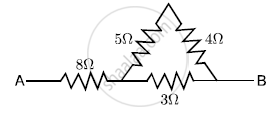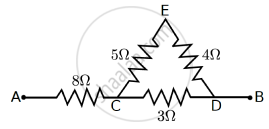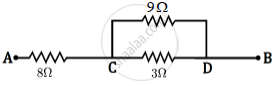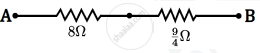# Calculate the Effective Resistance Across Ab ? - Physics

Short Note

Calculate the effective resistance across AB?#### Solution

5 Ω and 4Ω are in series∴ R1 = (5 + 4)Ω = 9ΩNow, 9Ω and 3Ω are in parallel
therefore R_2 = (9 xx 3)/(9+3)=9/4Ω
Now, 2 8Ω and R2 are in seriestherefore R_3 = 8 +9/4 =41/4 Ω= 10.25Ω

Concept: Ohm's Law
Is there an error in this question or solution?